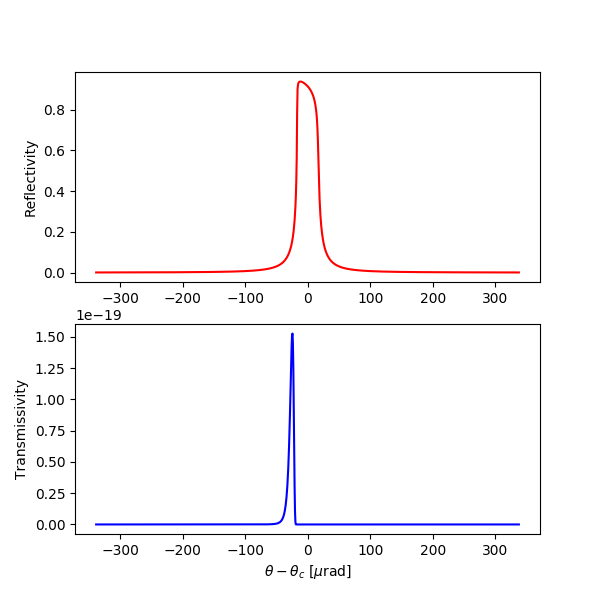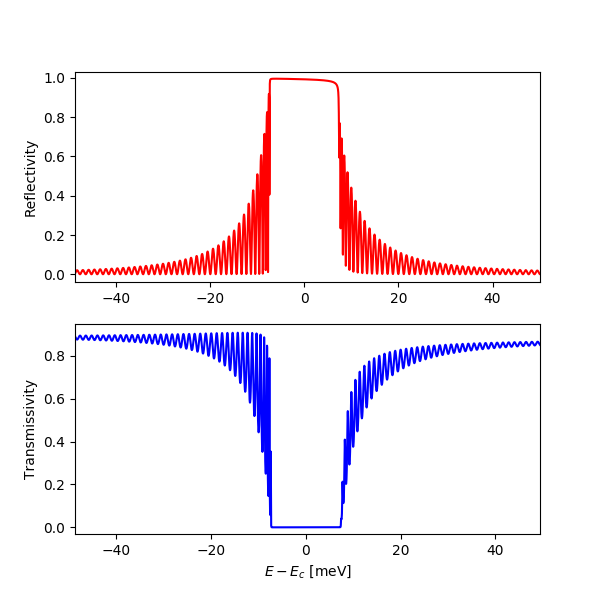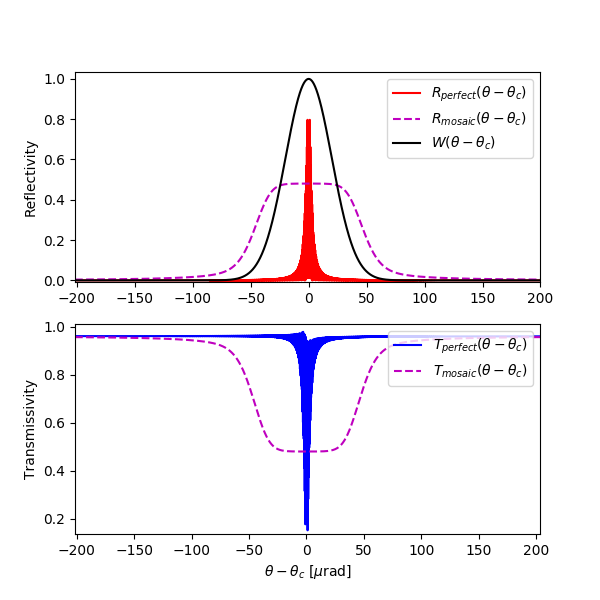dtxrd¶

x-ray diffraction calculator (dynamical theory of x-ray diffraction)

author: Stanislav Stoupin

SYNOPSIS¶

dtxrd [options] crystal h k l eta phi T d ["a" | "e"] [theta | Ex]

DESCRIPTION¶

Calculates parameters of a given crystal reflection for a monochromatic incident wave using dynamical theory of x-ray diffraction for perfect crystals in the 2-beam approximation

For a brief summary run:

dtxrd -h

INPUT PARAMETERS¶

crystal:

available crystal models: C (diamond), Si (silicon), Ge (germanium), GaN (wurtzite), SiC-4H, SiC-6H, SiO2 (quartz), Al2O3 (sapphire)

h k l:

Miller indicies of a chosen reflection

eta:

asymmetry angle ($$\eta$$ [degrees])

phi:

azimuthal angle of incidence ($$\phi$$ [degrees])

T:

crystal temperature [K]

d:

crystal thickness [mm]

flag:
flag description
a perform calculation at a given glancing angle of incidence theta
e perform calculation at a given photon energy Ex
theta:

glancing angle of incidence, theta ($$\theta$$)

Ex:

photon energy, Ex ($$E_{\mathrm X}$$)

OPTIONS¶

-v, --version:
show program version
-h, --help:
show summary of options.
-o FILENAME, --output FILENAME:
write results to file (default to stdout)
-w FILENAME, --write FILENAME:
write data to file (default: no action)
-p, --pi:$$\pi$$ polarization for the incident wave (default: $$\sigma$$ polarization)
-c CONST, --conv CONST:
convolve the reflectivity curve with a virtual instrument resolution function with FWHM = CONST [urad], plot the result and and report the resulting width of the convoluted curve
-s CONST, --syield CONST:
calculate shape of the secondary yield curve (e.g., photoelectrons) with escape depth CONST [Angstrom]
-z STRING, --zblock STRING:
calculate reflectivity/transmissivity curves for a mosaic crystal (uncorrelated block model) with STRING = 't s', where t is the block thickness [um] and s is the standard deviation of misorientation [urad] (assuming Gaussian distribution)
-n CONST, --nsteps CONST:
CONST - number of points in the angular/energy interval (default: 1000)

OUTPUT PARAMETERS¶

Basic parameters of the chosen h k l reflection:

d[A]: $$d$$ [Angstrom] interplanar distance (d-spacing) of the chosen h k l reflection $$E_B = \frac{hc}{2d}$$ [keV] Bragg energy $$\theta_R$$ [degrees] incident glancing angle for the exact backscattering (a wave with photon energy $$E_R$$ incident at this angle is reflected exactly backwards) $$E_R$$ [keV] photon energy for the exact backscattering $$b_{H}$$ asymmetry factor in the chosen scattering geometry for symmetric reflection $$\eta = 0$$ and $$b_{H} = - 1$$

Susceptibilities and refraction corrections:

chi_{0}: $$\chi_0$$ susceptibility $$\chi_{H}$$ susceptibility $$\chi_{\bar{H}}$$ susceptibility $$\omega_{H}^s$$ refraction correction for symmetric reflection $$\omega_{H} = \omega_{H}^s \frac{b_{H}-1}{2b_{H}}$$ refraction correction for the chosen reflectoin

Central energy and angle:

Ec[keV]: $$E_c$$ [keV] central energy of the chosen reflection $$\theta_c$$ [deg] central glancing angle of incidence of the chosen reflection

Energy intrinsic (Darwin) widths (thick non-absorbing crystal) at fixed glancing angle of incidence $$\theta_c$$:

eps_s: $$\varepsilon^s$$ relative energy width of symmetric h k l reflection (same for entrance and exit) $$\varepsilon$$ relative entrance energy width of the chosen h k l reflection $$\varepsilon'$$ relative exit energy width of the chosen h k l reflection $$\Delta E^s$$ [meV] absolute energy width of symmetric h k l reflection (same for entrance and exit) $$\Delta E$$ [meV] absolute entrance energy width of the chosen h k l reflection $$\Delta E'$$ [meV] absolute exit energy width of symmetric reflection

Angular intrinsic (Darwin) widths (thick non-absorbing crystal) at fixed photon energy $$E_c$$:

dth_s[urad]: $$\Delta \theta^s$$ [microradian] angular width of the symmetric h k l reflection (same for entrance and exit) $$\Delta \theta$$ [microradian] angular entrance width of the chosen h k l reflection $$\Delta \theta'$$ [microradian] angular exit width of the chosen h k l reflection

Additional characteristics of the chosen h k l reflection:

$$\frac{dE}{d\theta}$$ [meV/microradian] tangent of the Bragg's Law
Dr[urad/meV]:$$D_r$$ [microradian/meV] intrinsic angular dispersion rate of the chosen h k l reflection
de[um]:$$d_e$$ [micrometer] extinction length of the chosen h k l reflection

Reflectivity and Transmissivity:

Rc[%]: $$R_c$$ [%] reflectivity at center $$T_c$$ [%] transmissivity at center

EXAMPLES¶

A rocking curve of the symmetric Si 111 reflection (Bragg case, 1-mm-thick crystal at 300 K)

dtxrd Si 1 1 1 0 0 300 1 e 8A rocking curve of the symmetric diamond 220 reflection (Laue case, 0.1-mm-thick crystal plate at 300 K)

dtxrd C 2 2 0 90 0 300 0.1 e 12Reflectivity curve of the diamond 008 reflection in exact backscattering (0.5-mm-thick crystal plate at 300 K). Accurate sampling of the thickness oscillations is achieved using 10000 points.

dtxrd -n 10000 C 0 0 8 0 0 300 0.5 a 90Rocking curve of the diamond 220 reflection (0.5-mm-thick crystal plate at 300 K at 20 keV). Reflectivity/transmissivity of a perfect crystal compared with those of the mosaic crystal with 10 um block size having misorientation of 20 microradian r.m.s. (uncorrelated block model)

dtxrd -n 10000 -z '10 20' C 2 2 0 90 0 300 0.5 e 20Note: reflectivity for a mosaic crystal in backscattering has not been implemented yet